# RD Sharma Solutions For Class 12 Maths Exercise 3.4 Chapter 3 Binary Operations

RD Sharma Solutions for Class 12 Maths Exercise 3.4 Chapter 3 Binary Operations is provided here for students to study and excel in their main exams. This exercise deals with the inverse of an element of binary operations. It mainly consists of a set of examples before the exercise problems to make it possible for students to solve questions effortlessly. Students can access exercise-wise solutions in PDF format to solve problems of RD Sharma textbook easily. It improves problem-solving abilities of students, which are important from the exam point of view. RD Sharma Solutions for Class 12 Maths Chapter 3 Binary Operations Exercise 3.4 PDF are available here.

## Download the PDF of RD Sharma Solutions For Class 12 Maths Chapter 3 – Binary Operations Exercise 3.4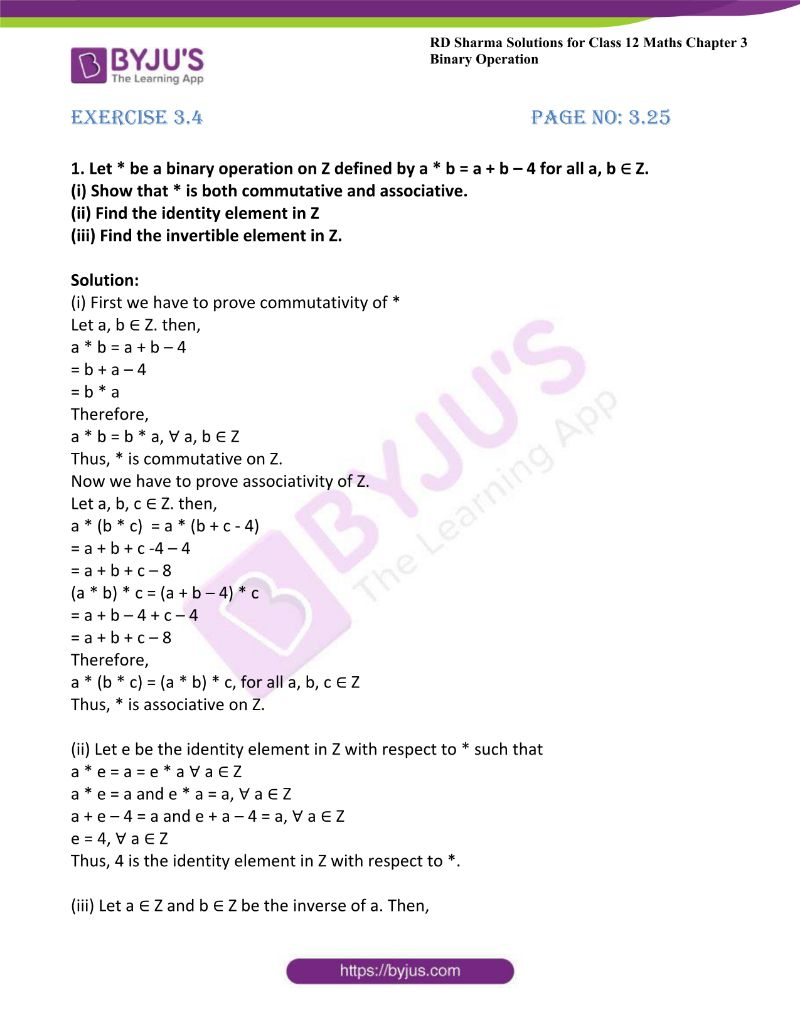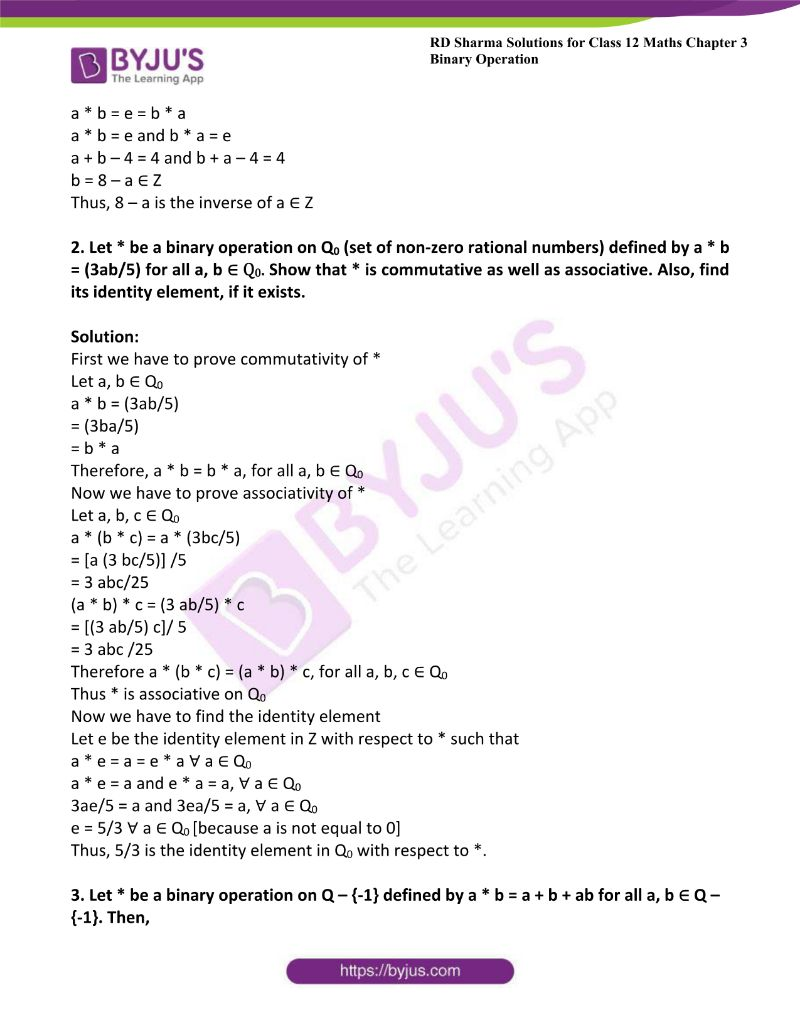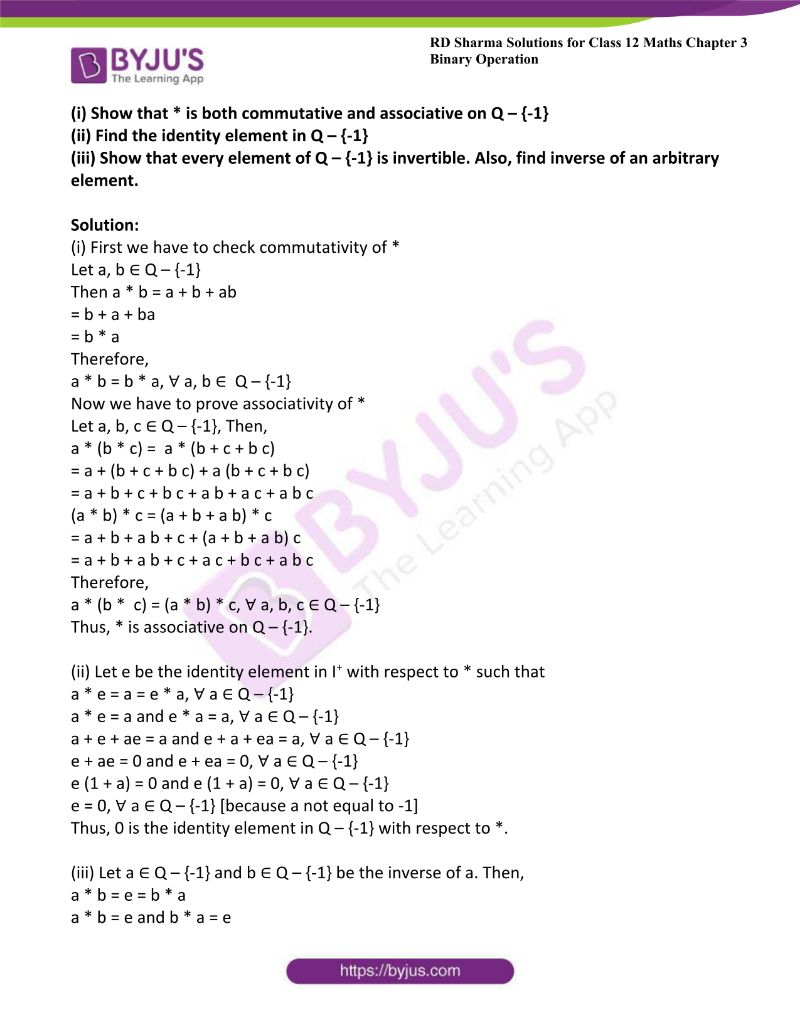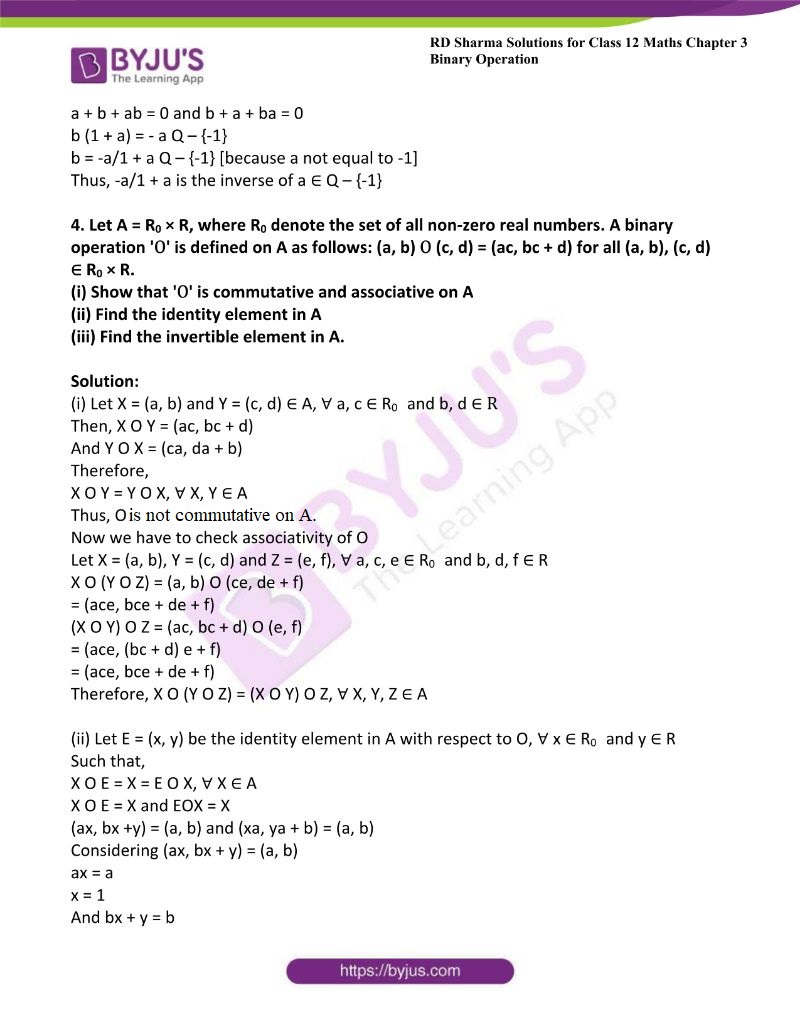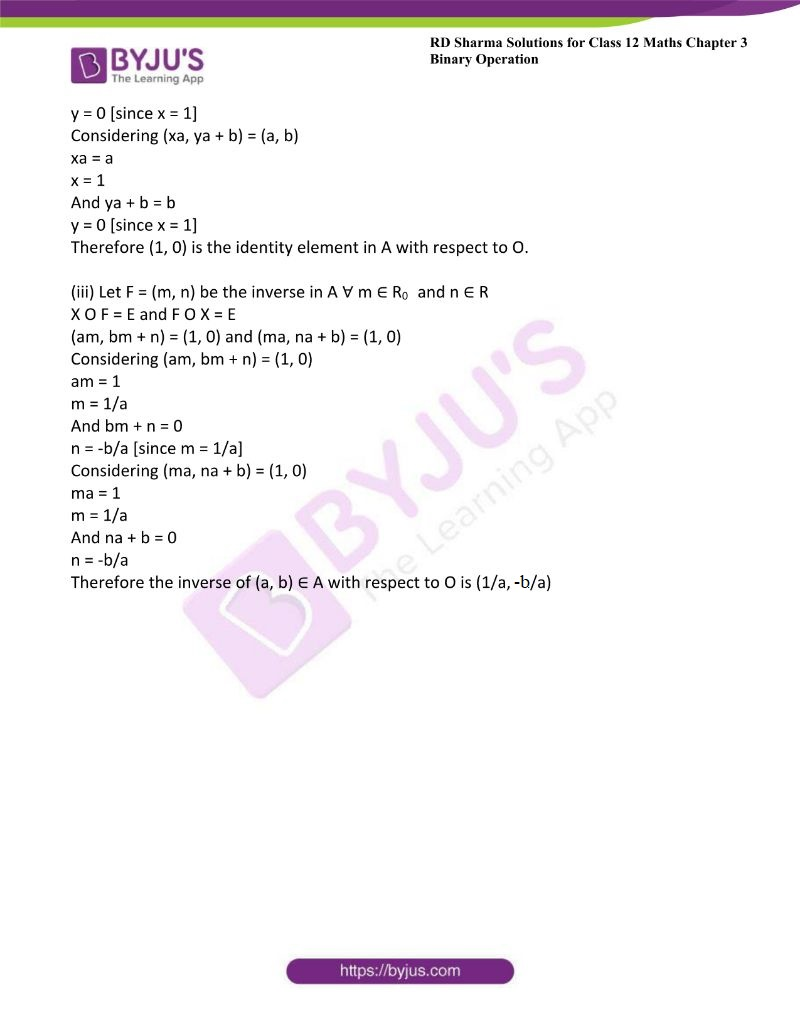### Access Other Exercises of RD Sharma Solutions For Class 12 Maths Chapter 3 – Binary Operations

Exercise 3.1 Solutions

Exercise 3.2 Solutions

Exercise 3.3 Solutions

Exercise 3.5 Solutions

### Access RD Sharma Solutions For Class 12 Maths Chapter 3 – Binary Operations Exercise 3.4

1. Let * be a binary operation on Z defined by a * b = a + b – 4 for all a, b ∈ Z.

(i) Show that * is both commutative and associative.

(ii) Find the identity element in Z

(iii) Find the invertible element in Z.

Solution:

(i) First we have to prove commutativity of *

Let a, b ∈ Z. then,

a * b = a + b – 4

= b + a – 4

= b * a

Therefore,

a * b = b * a, ∀ a, b ∈ Z

Thus, * is commutative on Z.

Now we have to prove associativity of Z.

Let a, b, c ∈ Z. then,

a * (b * c) = a * (b + c – 4)

= a + b + c -4 – 4

= a + b + c – 8

(a * b) * c = (a + b – 4) * c

= a + b – 4 + c – 4

= a + b + c – 8

Therefore,

a * (b * c) = (a * b) * c, for all a, b, c ∈ Z

Thus, * is associative on Z.

(ii) Let e be the identity element in Z with respect to * such that

a * e = a = e * a ∀ a ∈ Z

a * e = a and e * a = a, ∀ a ∈ Z

a + e – 4 = a and e + a – 4 = a, ∀ a ∈ Z

e = 4, ∀ a ∈ Z

Thus, 4 is the identity element in Z with respect to *.

(iii) Let a ∈ Z and b ∈ Z be the inverse of a. Then,

a * b = e = b * a

a * b = e and b * a = e

a + b – 4 = 4 and b + a – 4 = 4

b = 8 – a ∈ Z

Thus, 8 – a is the inverse of a ∈ Z

2. Let * be a binary operation on Q0 (set of non-zero rational numbers) defined by a * b = (3ab/5) for all a, b ∈ Q0. Show that * is commutative as well as associative. Also, find its identity element, if it exists.

Solution:

First we have to prove commutativity of *

Let a, b ∈ Q0

a * b = (3ab/5)

= (3ba/5)

= b * a

Therefore, a * b = b * a, for all a, b ∈ Q0

Now we have to prove associativity of *

Let a, b, c ∈ Q0

a * (b * c) = a * (3bc/5)

= [a (3 bc/5)] /5

= 3 abc/25

(a * b) * c = (3 ab/5) * c

= [(3 ab/5) c]/ 5

= 3 abc /25

Therefore a * (b * c) = (a * b) * c, for all a, b, c ∈ Q0

Thus * is associative on Q0

Now we have to find the identity element

Let e be the identity element in Z with respect to * such that

a * e = a = e * a ∀ a ∈ Q0

a * e = a and e * a = a, ∀ a ∈ Q0

3ae/5 = a and 3ea/5 = a, ∀ a ∈ Q0

e = 5/3 ∀ a ∈ Q0 [because a is not equal to 0]

Thus, 5/3 is the identity element in Q0 with respect to *.

3. Let * be a binary operation on Q – {-1} defined by a * b = a + b + ab for all a, b ∈ Q – {-1}. Then,

(i) Show that * is both commutative and associative on Q – {-1}

(ii) Find the identity element in Q – {-1}

(iii) Show that every element of Q – {-1} is invertible. Also, find inverse of an arbitrary element.

Solution:

(i) First we have to check commutativity of *

Let a, b ∈ Q – {-1}

Then a * b = a + b + ab

= b + a + ba

= b * a

Therefore,

a * b = b * a, ∀ a, b ∈ Q – {-1}

Now we have to prove associativity of *

Let a, b, c ∈ Q – {-1}, Then,

a * (b * c) = a * (b + c + b c)

= a + (b + c + b c) + a (b + c + b c)

= a + b + c + b c + a b + a c + a b c

(a * b) * c = (a + b + a b) * c

= a + b + a b + c + (a + b + a b) c

= a + b + a b + c + a c + b c + a b c

Therefore,

a * (b * c) = (a * b) * c, ∀ a, b, c ∈ Q – {-1}

Thus, * is associative on Q – {-1}.

(ii) Let e be the identity element in I+ with respect to * such that

a * e = a = e * a, ∀ a ∈ Q – {-1}

a * e = a and e * a = a, ∀ a ∈ Q – {-1}

a + e + ae = a and e + a + ea = a, ∀ a ∈ Q – {-1}

e + ae = 0 and e + ea = 0, ∀ a ∈ Q – {-1}

e (1 + a) = 0 and e (1 + a) = 0, ∀ a ∈ Q – {-1}

e = 0, ∀ a ∈ Q – {-1} [because a not equal to -1]

Thus, 0 is the identity element in Q – {-1} with respect to *.

(iii) Let a ∈ Q – {-1} and b ∈ Q – {-1} be the inverse of a. Then,

a * b = e = b * a

a * b = e and b * a = e

a + b + ab = 0 and b + a + ba = 0

b (1 + a) = – a Q – {-1}

b = -a/1 + a Q – {-1} [because a not equal to -1]

Thus, -a/1 + a is the inverse of a ∈ Q – {-1}

4. Let A = R0 × R, where R0 denote the set of all non-zero real numbers. A binary operation ‘O’ is defined on A as follows: (a, b) O (c, d) = (ac, bc + d) for all (a, b), (c, d) ∈ R0 × R.

(i) Show that ‘O’ is commutative and associative on A

(ii) Find the identity element in A

(iii) Find the invertible element in A.

Solution:

(i) Let X = (a, b) and Y = (c, d) ∈ A, ∀ a, c ∈ R0 and b, d ∈ R

Then, X O Y = (ac, bc + d)

And Y O X = (ca, da + b)

Therefore,

X O Y = Y O X, ∀ X, Y ∈ A

Thus, O is not commutative on A.

Now we have to check associativity of O

Let X = (a, b), Y = (c, d) and Z = (e, f), ∀ a, c, e ∈ R0 and b, d, f ∈ R

X O (Y O Z) = (a, b) O (ce, de + f)

= (ace, bce + de + f)

(X O Y) O Z = (ac, bc + d) O (e, f)

= (ace, (bc + d) e + f)

= (ace, bce + de + f)

Therefore, X O (Y O Z) = (X O Y) O Z, ∀ X, Y, Z ∈ A

(ii) Let E = (x, y) be the identity element in A with respect to O, ∀ x ∈ R0 and y ∈ R

Such that,

X O E = X = E O X, ∀ X ∈ A

X O E = X and EOX = X

(ax, bx +y) = (a, b) and (xa, ya + b) = (a, b)

Considering (ax, bx + y) = (a, b)

ax = a

x = 1

And bx + y = b

y = 0 [since x = 1]

Considering (xa, ya + b) = (a, b)

xa = a

x = 1

And ya + b = b

y = 0 [since x = 1]

Therefore (1, 0) is the identity element in A with respect to O.

(iii) Let F = (m, n) be the inverse in A ∀ m ∈ R0 and n ∈ R

X O F = E and F O X = E

(am, bm + n) = (1, 0) and (ma, na + b) = (1, 0)

Considering (am, bm + n) = (1, 0)

am = 1

m = 1/a

And bm + n = 0

n = -b/a [since m = 1/a]

Considering (ma, na + b) = (1, 0)

ma = 1

m = 1/a

And na + b = 0

n = -b/a

Therefore the inverse of (a, b) ∈ A with respect to O is (1/a, -b/a)